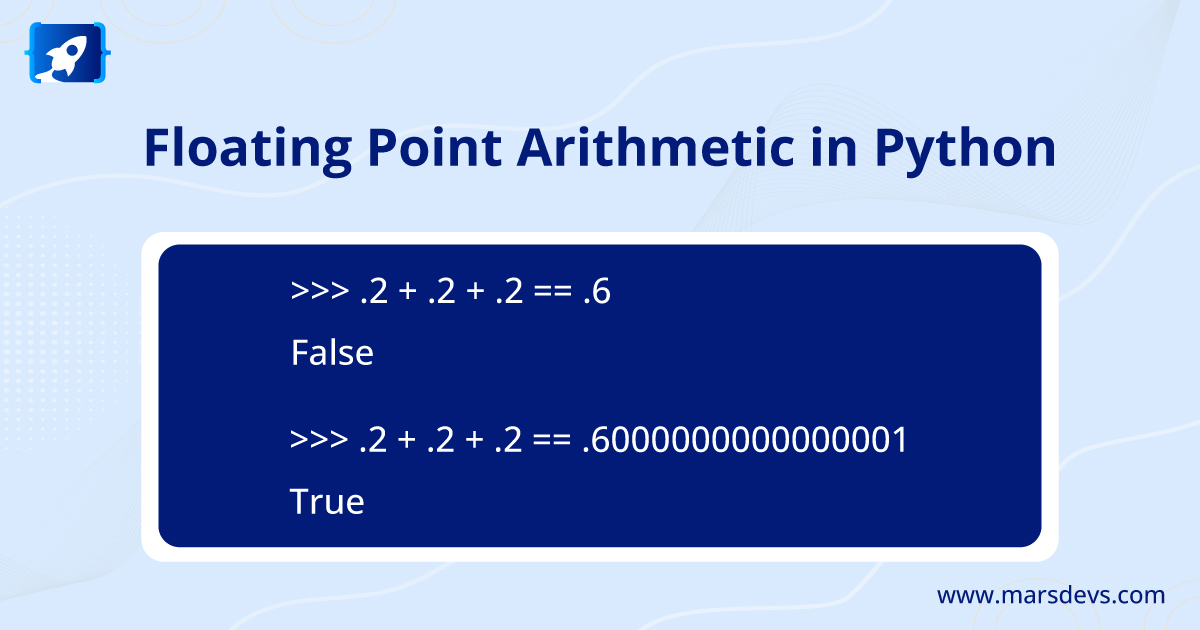# MarsDevs introduces you to Floating Point ArithmeticIn this article, MarsDevs will present to you what Floating point numbers are, how they are used in arithmetic in Python, and their issues and limitations.

### Introduction

In computer hardware, floating-point numbers are represented as base 2 (binary) fractions.

Example

The decimal fraction 0.125 has the value 1/10 + 2/100 + 5/1000, and the binary fraction 0.001 has the value 0/2 + 0/4 + 1/8. They have similar values, the only real difference is in their bases.

But not all fractions can be represented exactly as binary fractions. Then you can really only approximate by binary floating-point numbers stored in the computer.

Example

Let's look at this example in base 10. If you wanted to represent 1/9, the approximate value would be 0.1111111111111... in base 10. The value of 1/9 has an infinite number of 1's after the decimal point.

You can take 0.1 or better 0.11 or better 0.111 to represent in the machine. No matter how many digits you choose to write, the result will never be exactly 1/9, but a better approximation of 1/9.

Therefore, you cannot represent exactly 1/9 in a computer or machine, but you can represent its approximate value using a floating-point representation technique.

### Floating-Point Representation Technique

By choosing any finite number of bits. The most popular representation nowadays is using a binary fraction with the numerator using the first 53 bits starting from the most significant bit and with the denominator as a power of two.

Python simply prints a decimal approximation to the actual decimal value of the binary approximation stored by the machine. Otherwise, on most machines, it prints the entire value in 53 bits.

Example

The decimal value of 0.1 will be in 53 bits,

But it has more digits than most people find useful, so in Python print a rounded value instead.

Example

The decimal value of 0.1 in Python, normally,

Example

Similarly, it also has more digits than most people find useful, so in Python print a rounded value instead.

Example

Note that, even though the printed result looks like an exact value of 1/9, the actual stored value is the closest representable binary fraction.

### Issues

There can be the same representation for different numbers.

Example

Python's built-in repr() function will select 0.1000000000000000001 containing 17 significant digits. The smallest of these only displays 0.1.

It is in the nature of the binary floating-point representation that your hardware supports floating-point arithmetic.

Example

As you noticed there can be the same representation for different decimal values, so the following example is returning false.

Because 0.1 is not exactly 1/10 and 0.3 cannot get any closer to the exact value of 3/10. So, 0.1 + 0.1 + 0.1 may not yield exactly 0.3. So pre-rounding with the round() function may not help here.

But post-rounding with the round() function can help here so that results with exact values become comparable.

### Modules to exact decimal representation

For use cases that require exact decimal representation

• Using the decimal module
It implements decimal arithmetic suitable for accounting applications and high-precision applications.
• Using the fractions module
It implements arithmetic based on rational numbers, so numbers such as 1/9 can be represented accurately.

The NumPy package and many other packages for mathematical and statistical operations can also help with floating point number representation and arithmetic.

### (1). float.as_integer_ratio() method

The float.as_integer_ratio() method expresses the value of a float as a fraction. It tries to find the numerator and denominator of the ratio of the given fraction.

Example

For which

### (2). float.hex() method

The float.hex() method is used to express a float in hexadecimal (base 16). It gives the exact value stored by your machine.

Example

For which

Since the representation is exact, it can be ported to different versions of Python Exchange with other languages that support the same format.

### (3). math.fsum() method

The math.fsum() method helps reduce precision loss during summation. It tracks "lost digits" as values are added to the running total.

Example

## Representation Error

Representation error occurs when decimal fractions cannot be represented exactly as binary (base 2) fractions. This is the main reason why many programming languages do not display the exact decimal numbers you expect.

IEEE-754 floating point arithmetic is used in almost all computers, and Python supports IEEE-754 "double precision" floats as well. It is 53 bits accurate.

Example

1/10 is not exactly representable as a binary fraction. To round 0.1 to the nearest fraction, it can be in the form J/2**N where J is an integer with exactly 53 bits.

That means,

1 / 10 ~= J / (2**N)

J ~= 2**N / 10

Therefore,

Because J has exactly 53 bits (is>= 2**5 2 but < 2**53), and the best value for N is 56.

Hence,

The approximation to 1/10 in 754 double precision is

7205759403792794 / 2 ** 56

3602879701896397 / 2 ** 55  # Dividing both the numerator and denominator by two

It's not exactly 1/10 with or without the target. If you round, it will be slightly larger than 1/10, and if you don't, the quotient will be slightly smaller than 1/10. The above number has been rounded off. Therefore, it cannot be exactly 1/10 in any case.

0.1 * 2 ** 55

If we multiply by 10**55, then the value out to 55 decimal digits

If we round up in Python

By using decimal and fractions modules